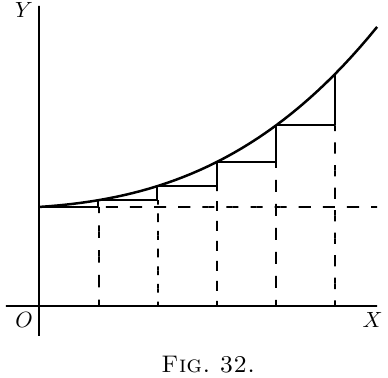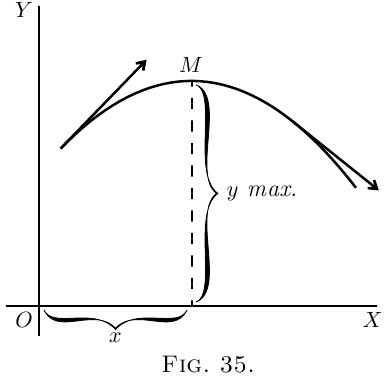# Curvature of Curves

Returning to the process of successive differentiation, it may be asked: Why does anybody want to differentiate twice over? We know that when the variable quantities are space and time, by differentiating twice over we get the acceleration of a moving body, and that in the geometrical interpretation, as applied to curves, $\dfrac{dy}{dx}$ means the slope of the curve. But what can $\dfrac{d^2 y}{dx^2}$ mean in this case? Clearly it means the rate (per unit of length $x$) at which the slope is changing–in brief, it is a measure of the curvature of the slope.Here, $\dfrac{dy}{dx}$ is of constant value.

Suppose, however, a case in which, like Figure 32, the slope itself is getting greater upwards, then $\dfrac{d\left(\dfrac{dy}{dx}\right)}{dx}$, that is, $\dfrac{d^2y}{dx^2}$, will be positive.

If the slope is becoming less as you go to the right (as in Figure 14), or as in Figure 33, then, even though the curve may be going upward, since the change is such as to diminish its slope, its $\dfrac{d^2y}{dx^2}$ will be negative.The reason of it ought to be quite evident. Think of any curve that has a minimum point in it (like Figure 15), or like Figure 34, where the point of minimum $y$ is marked $M$, and the curve is concave upwards. To the left of $M$ the slope is downward, that is, negative, and is getting less negative. To the right of $M$ the slope has become upward, and is getting more and more upward. Clearly the change of slope as the curve passes through $M$ is such that $\dfrac{d^2y}{dx^2}$ is positive, for its operation, as $x$ increases toward the right, is to convert a downward slope into an upward one.

Similarly, consider any curve that has a maximum point in it (like Figure 16), or like Figure 35, where the curve is convex, and the maximum point is marked $M$. In this case, as the curve passes through $M$ from left to right, its upward slope is converted into a downward or negative slope, so that in this case the “slope of the slope” $\dfrac{d^2y}{dx^2}$ is negative.

Go back now to the examples of the last chapter and verify in this way the conclusions arrived at as to whether in any particular case there is a maximum or a minimum. You will find below a few worked out examples.

(1) Find the maximum or minimum of \begin{align*} \text{(a)}\quad y &= 4x^2-9x-6; \qquad \text{(b)}\quad y = 6 + 9x-4x^2; \\ \end{align*} and ascertain if it be a maximum or a minimum in each case. \begin{align*} \text{(a)}\quad \dfrac{dy}{dx} &= 8x-9=0;\quad x=1\tfrac{1}{8},\quad \text{and } y = -11.065.\\ \dfrac{d^2y}{dx^2} &= 8;\quad \text{it is $+$; hence it is a minimum.} \\ \text{(b)}\quad {\dfrac{dy}{dx}} &= 9-8x=0;\quad x = 1\tfrac{1}{8};\quad \text{and } y = +11.065.\\ \dfrac{d^2y}{dx^2} &= -8;\quad \text{it is $-$; hence it is a maximum.} \end{align*}

(2) Find the maxima and minima of the function $y = x^3-3x+16$. \begin{align*} \dfrac{dy}{dx} &= 3x^2 - 3 = 0;\quad x^2 = 1;\quad \text{and } x = ±1.\\ \dfrac{d^2y}{dx^2} &= 6x;\quad \text{for $x = 1$; it is $+$}; \end{align*} hence $x=1$ corresponds to a minimum $y=14$. For $x=-1$ it is $-$; hence $x=-1$ corresponds to a maximum $y=+18$.

(3) Find the maxima and minima of $y=\dfrac{x-1}{x^2+2}$. $\frac{dy}{dx} = \frac{(x^2+2) × 1 - (x-1) × 2x}{(x^2+2)^2} = \frac{2x - x^2 + 2}{(x^2 + 2)^2} = 0;$ or $x^2 - 2x - 2 = 0$, whose solutions are $x =+2.73$ and $x=-0.73$. \begin{align*} \dfrac{d^2y}{dx^2} &= - \frac{(x^2 + 2)^2 × (2x-2) - (x^2 - 2x - 2)(4x^3 + 8x)}{(x^2 + 2)^4} \\ &= - \frac{2x^5 - 6x^4 - 8x^3 - 8x^2 - 24x + 8}{(x^2 + 2)^4}. \end{align*}

The denominator is always positive, so it is sufficient to ascertain the sign of the numerator.

If we put $x = 2.73$, the numerator is negative; the maximum, $y = 0.183$.

If we put $x=-0.73$, the numerator is positive; the minimum, $y=-0.683$.

(4) The expense $C$ of handling the products of a certain factory varies with the weekly output $P$ according to the relation $C = aP + \dfrac{b}{c+P} + d$, where $a$, $b$, $c$, $d$ are positive constants. For what output will the expense be least? $\dfrac{dC}{dP} = a - \frac{b}{(c+P)^2} = 0\quad \text{for maximum or minimum;}$ hence $a = \dfrac{b}{(c+P)^2}$ and $P = ±\sqrt{\dfrac{b}{a}} - c$.

As the output cannot be negative, $P=+\sqrt{\dfrac{b}{a}} - c$. \begin{align*} Now \frac{d^2C}{dP^2} &= + \frac{b(2c + 2P)}{(c + P)^4}, \end{align*} which is positive for all the values of $P$; hence $P = +\sqrt{\dfrac{b}{a}} - c$ corresponds to a minimum.

(5) The total cost per hour $C$ of lighting a building with $N$ lamps of a certain kind is $C = N\left(\frac{C_l}{t} + \frac{EPC_e}{1000}\right),$ where $E$ is the commercial efficiency (watts per candle), $P$ is the candle power of each lamp,
$t$ is the average life of each lamp in hours,
$C_l =$ cost of renewal in pence per hour of use,
$C_e =$ cost of energy per $1000$ watts per hour.

Moreover, the relation connecting the average life of a lamp with the commercial efficiency at which it is run is approximately $t = mE^n$, where $m$ and $n$ are constants depending on the kind of lamp.

Find the commercial efficiency for which the total cost of lighting will be least. \begin{align*} \text{ We have}\; C &= N\left(\frac{C_l}{m} E^{-n} + \frac{PC_e}{1000} E\right), \\ \dfrac{dC}{dE} &= \frac{PC_e}{1000} - \frac{nC_l}{m} E^{-(n+1)} = 0 \end{align*} for maximum or minimum. $E^{n+1} = \frac{1000 × nC_l}{mPC_e}\quad \text{and}\quad E = \sqrt[n+1]{\frac{1000 × nC_l}{mPC_e}}.$

This is clearly for minimum, since $\frac{d^2C}{dE^2} = (n + 1) \frac{nC_l}{m} E^{-(n+2)},$ which is positive for a positive value of $E$.

For a particular type of $16$ candle-power lamps, $C_l= 17$ pence, $C_e=5$ pence; and it was found that $m=10$ and $n=3.6$. $E = \sqrt[4.6]{\frac{1000 × 3.6 × 17}{10 × 16 × 5}} = 2.6\text{ watts per candle-power}.$

### Exercises X

(You are advised to plot the graph of any numerical example.) (1) Find the maxima and minima of $y = x^3 + x^2 - 10x + 8.$

(2) Given $y = \dfrac{b}{a}x - cx^2$, find expressions for $\dfrac{dy}{dx}$, and for $\dfrac{d^2y}{dx^2}$, also find the value of $x$ which makes $y$ a maximum or a minimum, and show whether it is maximum or minimum.

(3) Find how many maxima and how many minima there are in the curve, the equation to which is $y = 1 - \frac{x^2}{2} + \frac{x^4}{24};$ and how many in that of which the equation is $y = 1 - \frac{x^2}{2} + \frac{x^4}{24} - \frac{x^6}{720}.$

(4) Find the maxima and minima of $y=2x+1+\frac{5}{x^2}.$

(5) Find the maxima and minima of $y=\frac{3}{x^2+x+1}.$

(6) Find the maxima and minima of $y=\frac{5x}{2+x^2}.$

(7) Find the maxima and minima of $y=\frac{3x}{x^2-3} + \frac{x}{2} + 5.$

(8) Divide a number $N$ into two parts in such a way that three times the square of one part plus twice the square of the other part shall be a minimum.

(9) The efficiency $u$ of an electric generator at different values of output $x$ is expressed by the general equation: $u=\frac{x}{a+bx+cx^2};$ where $a$ is a constant depending chiefly on the energy losses in the iron and $c$ a constant depending chiefly on the resistance of the copper parts. Find an expression for that value of the output at which the efficiency will be a maximum.

(10) Suppose it to be known that consumption of coal by a certain steamer may be represented by the formula $y = 0.3 + 0.001v^3$; where $y$ is the number of tons of coal burned per hour and $v$ is the speed expressed in nautical miles per hour. The cost of wages, interest on capital, and depreciation of that ship are together equal, per hour, to the cost of $1$ ton of coal. What speed will make the total cost of a voyage of $1000$ nautical miles a minimum? And, if coal costs $10$ shillings per ton, what will that minimum cost of the voyage amount to?

(11) Find the maxima and minima of $y = ±\frac{x}{6}\sqrt{x(10-x)}.$

(12) Find the maxima and minima of $y= 4x^3 - x^2 - 2x + 1.$

### Answers

(1) Max.: $x = -2.19$, $y = 24.19$; min.:, $x = 1.52$, $y = -1.38$.

(2) $\dfrac{dy}{dx} = \dfrac{b}{a} - 2cx$; $\dfrac{d^2 y}{dx^2} = -2c$; $x = \dfrac{b}{2ac}$ (a maximum).

(3) (a ) One maximum and two minima. (b ) One maximum. ($x = 0$; other points unreal.)

(4) Min.: $x = 1.71$, $y = 6.14$.

(5) Max: $x = -.5$, $y = 4$.

(6) Max.: $x = 1.414$, $y = 1.7675$. Min.: $x = -1.414$, $y = 1.7675$.

(7) Max.: $x = -3.565$, $y = 2.12$. Min.: $x = +3.565$, $y = 7.88$.

(8) $0.4N$, $0.6N$.

(9) $x = \sqrt{\dfrac{a}{c}}$.

(10) Speed $8.66$ nautical miles per hour. Time taken $115.47$ hours. Minimum cost £$112$. $12$s .

(12) Min.: $x = \frac{1}{2}$, $y= 0.25$; max.: $x = - \frac{1}{3}$, $y= 1.408$.

Next →
Main Page ↑